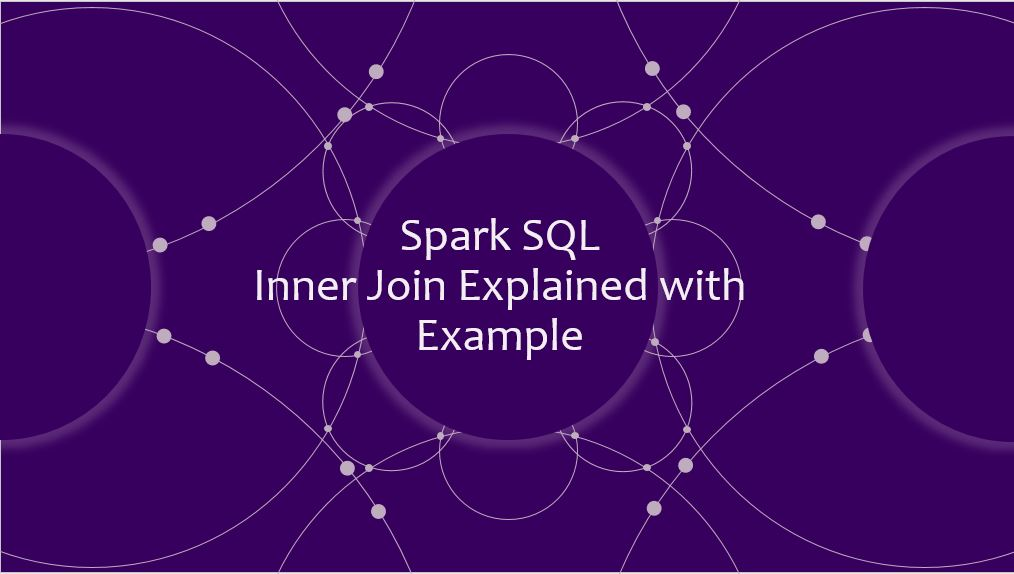# Spark Join Multiple DataFrames | Tables

Spark supports joining multiple (two or more) DataFrames, In this article, you will learn how to use a Join on multiple DataFrames using Spark SQL expression(on tables) and Join operator with Scala example. Also, you will learn different ways to provide Join condition.

In order to explain join with multiple tables, we will use Inner join, this is the default join in Spark and it’s mostly used, this joins two DataFrames/Datasets on key columns, and where keys don’t match the rows get dropped from both datasets.

Before we jump into Spark Join examples, first, let’s create an `"emp"` , `"dept"`, `"address"` DataFrame tables.

Emp Table

``````
val emp = Seq((1,"Smith","10"),
(2,"Rose","20"),
(3,"Williams","10"),
(4,"Jones","10"),
(5,"Brown","40"),
(6,"Brown","50")
)
val empColumns = Seq("emp_id","name","emp_dept_id")
import spark.sqlContext.implicits._
val empDF = emp.toDF(empColumns:_*)
empDF.show(false)
``````

Yields below output.

``````
+------+--------+-----------+
|emp_id|name    |emp_dept_id|
+------+--------+-----------+
|1     |Smith   |10         |
|2     |Rose    |20         |
|3     |Williams|10         |
|4     |Jones   |10         |
|5     |Brown   |40         |
|6     |Brown   |50         |
+------+--------+-----------+
``````

Dept Table

``````
val dept = Seq(("Finance",10),
("Marketing",20),
("Sales",30),
("IT",40)
)
val deptColumns = Seq("dept_name","dept_id")
val deptDF = dept.toDF(deptColumns:_*)
deptDF.show(false)
``````

Yields below output.

``````
+---------+-------+
|dept_name|dept_id|
+---------+-------+
|Finance  |10     |
|Marketing|20     |
|Sales    |30     |
|IT       |40     |
+---------+-------+
``````

``````
val address = Seq((1,"1523 Main St","SFO","CA"),
(2,"3453 Orange St","SFO","NY"),
(3,"34 Warner St","Jersey","NJ"),
(4,"221 Cavalier St","Newark","DE"),
(5,"789 Walnut St","Sandiago","CA")
)
``````

Yields below output.

``````
+------+---------------+--------+-----+
+------+---------------+--------+-----+
|1     |1523 Main St   |SFO     |CA   |
|2     |3453 Orange St |SFO     |NY   |
|3     |34 Warner St   |Jersey  |NJ   |
|4     |221 Cavalier St|Newark  |DE   |
|5     |789 Walnut St  |Sandiago|CA   |
+------+---------------+--------+-----+
``````

## Using Join operator

``````
join(right: Dataset[_], joinExprs: Column, joinType: String): DataFrame
join(right: Dataset[_]): DataFrame
``````

The first join syntax takes, takes `right` dataset, `joinExprs` and `joinType` as arguments and we use `joinExprs` to provide a join condition. second join syntax takes just dataset and joinExprs and it considers default join as `<a href="https://sparkbyexamples.com/spark/spark-sql-dataframe-join/#sql-inner-join">inner join</a>`. The rest of the article uses both syntaxes to join multiple Spark DataFrames.

``````
//Using Join expression
empDF.join(deptDF,empDF("emp_dept_id") === deptDF("dept_id"),"inner" )
.show(false)
``````

This joins all 3 tables and returns a new DataFrame with the below result.

``````
+------+--------+-----------+---------+-------+------+---------------+--------+-----+
+------+--------+-----------+---------+-------+------+---------------+--------+-----+
|1     |Smith   |10         |Finance  |10     |1     |1523 Main St   |SFO     |CA   |
|2     |Rose    |20         |Marketing|20     |2     |3453 Orange St |SFO     |NY   |
|3     |Williams|10         |Finance  |10     |3     |34 Warner St   |Jersey  |NJ   |
|4     |Jones   |10         |Finance  |10     |4     |221 Cavalier St|Newark  |DE   |
|5     |Brown   |40         |IT       |40     |5     |789 Walnut St  |Sandiago|CA   |
+------+--------+-----------+---------+-------+------+---------------+--------+-----+
``````

Alternatively, you can also use `Inner.sql` as jointype and to use this you should import `import org.apache.spark.sql.catalyst.plans.Inner`

``````
//Using Join expression
empDF.join(deptDF,empDF("emp_dept_id") === deptDF("dept_id"),Inner.sql )
.show(false)
``````

The rest of the article provides a spark Inner Join example using DataFrame `where()`, `filter()` operators and `spark.sql()`, all these examples provide the same output as above.

## Using Where to provide Join condition

Instead of using a join condition with `join()` operator, here, we use `where()` to provide an inner join condition.

``````
//Using where
empDF.join(deptDF).where(empDF("emp_dept_id") === deptDF("dept_id"))
.show(false)
``````

## Using Filter to provide Join condition

We can also use `filter()` to provide join condition for Spark Join operations

``````
//Using Filter
empDF.join(deptDF).filter(empDF("emp_dept_id") === deptDF("dept_id"))
.show(false)
``````

## Using SQL Expression

Here, we will use the native SQL syntax to do join on multiple tables, in order to use Native SQL syntax, first, we should create a temporary view for all our DataFrames and then use `spark.sql()` to execute the SQL expression.

``````
//Using SQL expression
empDF.createOrReplaceTempView("EMP")
deptDF.createOrReplaceTempView("DEPT")

spark.sql("select * from EMP e, DEPT d, ADD a " +
"where e.emp_dept_id == d.dept_id and e.emp_id == a.emp_id")
.show(false)
``````

## Source code of Spark Join on multiple DataFrames

``````
package com.sparkbyexamples.spark.dataframe.join

import org.apache.spark.sql.SparkSession

object JoinMultipleDataFrames extends App {

val spark: SparkSession = SparkSession.builder()
.master("local")
.appName("SparkByExamples.com")
.getOrCreate()

spark.sparkContext.setLogLevel("ERROR")

val emp = Seq((1,"Smith","10"),
(2,"Rose","20"),
(3,"Williams","10"),
(4,"Jones","10"),
(5,"Brown","40"),
(6,"Brown","50")
)
val empColumns = Seq("emp_id","name","emp_dept_id")
import spark.sqlContext.implicits._
val empDF = emp.toDF(empColumns:_*)
empDF.show(false)

val dept = Seq(("Finance",10),
("Marketing",20),
("Sales",30),
("IT",40)
)
val deptColumns = Seq("dept_name","dept_id")
val deptDF = dept.toDF(deptColumns:_*)
deptDF.show(false)

val address = Seq((1,"1523 Main St","SFO","CA"),
(2,"3453 Orange St","SFO","NY"),
(3,"34 Warner St","Jersey","NJ"),
(4,"221 Cavalier St","Newark","DE"),
(5,"789 Walnut St","Sandiago","CA")
)

//Using Join expression
empDF.join(deptDF,empDF("emp_dept_id") === deptDF("dept_id"),"inner" )
.show(false)

//Using where
empDF.join(deptDF).where(empDF("emp_dept_id") === deptDF("dept_id"))
.show(false)

//Using Filter
empDF.join(deptDF).filter(empDF("emp_dept_id") === deptDF("dept_id"))
.show(false)

//Using SQL expression
empDF.createOrReplaceTempView("EMP")
deptDF.createOrReplaceTempView("DEPT")

spark.sql("select * from EMP e, DEPT d, ADD a " +
"where e.emp_dept_id == d.dept_id and e.emp_id == a.emp_id")
.show(false)
}

``````

The complete example is available at GitHub project for reference.

## Conclusion

In this Spark article, you have learned how to join multiple DataFrames and tables(creating temporary views) with Scala example and also learned how to use conditions using where filter.

Thanks for reading. If you like it, please do share the article by following the below social links and any comments or suggestions are welcome in the comments sections!

Happy Learning !!

### NNK

SparkByExamples.com is a Big Data and Spark examples community page, all examples are simple and easy to understand and well tested in our development environment Read more ..